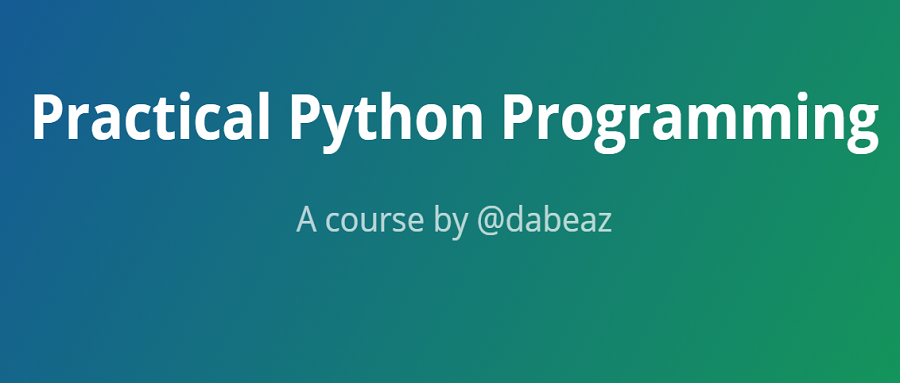# 翻译：《实用的 Python 编程》01_03_Numberscodists# 1.3 数字

### 数字类型

Python 有 4 种类型的数字：

• 布尔型

• 整型

• 浮点型

• 复数（虚数）

### 布尔型（bool）

a = Trueb = False

c = 4 + True # 5d = Falseif d == 0:    print('d is False')

### 整型（int）

a = 37b = -299392993727716627377128481812241231c = 0x7fa8      # Hexadecimald = 0o253       # Octale = 0b10001111  # Binary

x + y      Addx - y      Subtractx * y      Multiplyx / y      Divide (produces a float)x // y     Floor Divide (produces an integer)x % y      Modulo (remainder)x ** y     Powerx << n     Bit shift leftx >> n     Bit shift rightx & y      Bit-wise ANDx | y      Bit-wise ORx ^ y      Bit-wise XOR~x         Bit-wise NOTabs(x)     Absolute value

### 浮点型（float）

a = 37.45b = 4e5 # 4 x 10**5 or 400,000c = -1.345e-10

17 digits of precision

Exponent from -308 to 308

>>> a = 2.1 + 4.2>>> a == 6.3False>>> a6.300000000000001>>>

x + y      Addx - y      Subtractx * y      Multiplyx / y      Dividex // y     Floor Dividex % y      Modulox ** y     Powerabs(x)     Absolute Value

import matha = math.sqrt(x)b = math.sin(x)c = math.cos(x)d = math.tan(x)e = math.log(x)

### 比较

x < y      Less thanx <= y     Less than or equalx > y      Greater thanx >= y     Greater than or equalx == y     Equal tox != y     Not equal to

if b >= a and b <= c:    print('b is between a and c')if not (b < a or b > c):    print('b is still between a and c')

### 转换数字

a = int(x)    # Convert x to integerb = float(x)  # Convert x to float

>>> a = 3.14159>>> int(a)3>>> b = '3.14159' # It also works with strings containing numbers>>> float(b)3.14159>>>

## 习题

### 习题 1.7：戴夫的抵押贷款

# mortgage.pyprincipal = 500000.0rate = 0.05payment = 2684.11total_paid = 0.0while principal > 0:    principal = principal * (1+rate/12) - payment    total_paid = total_paid + paymentprint('Total paid', total_paid)

### 习题 1.9：制作一个额外的付款计算器

extra_payment_start_month = 61extra_payment_end_month = 108extra_payment = 1000

### 习题 1.10：制作表格

1 2684.11 499399.222 5368.22 498795.943 8052.33 498190.154 10736.44 497581.835 13420.55 496970.98...308 874705.88 3478.83309 877389.99 809.21310 880074.1 -1871.53Total paid 880074.1Months 310

### 习题 1.12：一个谜

int() 函数和 float() 函数可以将其它类型的数据转换为数字类型。示例：

>>> int("123")123>>> float("1.23")1.23>>>

>>> bool("False")True>>>### codists

Life is short, You need Python

## 评论